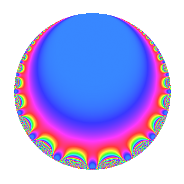Properties

 Label 16.3.cLevel 16 Weight 3 Character orbit c Rep. character $$\chi_{16}(15,\cdot)$$ Character field $$\Q$$ Dimension 1 Newforms 1 Sturm bound 6 Trace bound 0

Related objects

Defining parameters

 Level: $$N$$ = $$16 = 2^{4}$$ Weight: $$k$$ = $$3$$ Character orbit: $$[\chi]$$ = 16.c (of order $$2$$ and degree $$1$$) Character conductor: $$\operatorname{cond}(\chi)$$ = $$4$$ Character field: $$\Q$$ Newforms: $$1$$ Sturm bound: $$6$$ Trace bound: $$0$$

Dimensions

The following table gives the dimensions of various subspaces of $$M_{3}(16, [\chi])$$.

Total New Old
Modular forms 7 1 6
Cusp forms 1 1 0
Eisenstein series 6 0 6

Trace form

 $$q$$ $$\mathstrut -\mathstrut 6q^{5}$$ $$\mathstrut +\mathstrut 9q^{9}$$ $$\mathstrut +\mathstrut O(q^{10})$$ $$q$$ $$\mathstrut -\mathstrut 6q^{5}$$ $$\mathstrut +\mathstrut 9q^{9}$$ $$\mathstrut +\mathstrut 10q^{13}$$ $$\mathstrut -\mathstrut 30q^{17}$$ $$\mathstrut +\mathstrut 11q^{25}$$ $$\mathstrut +\mathstrut 42q^{29}$$ $$\mathstrut -\mathstrut 70q^{37}$$ $$\mathstrut +\mathstrut 18q^{41}$$ $$\mathstrut -\mathstrut 54q^{45}$$ $$\mathstrut +\mathstrut 49q^{49}$$ $$\mathstrut +\mathstrut 90q^{53}$$ $$\mathstrut -\mathstrut 22q^{61}$$ $$\mathstrut -\mathstrut 60q^{65}$$ $$\mathstrut -\mathstrut 110q^{73}$$ $$\mathstrut +\mathstrut 81q^{81}$$ $$\mathstrut +\mathstrut 180q^{85}$$ $$\mathstrut -\mathstrut 78q^{89}$$ $$\mathstrut +\mathstrut 130q^{97}$$ $$\mathstrut +\mathstrut O(q^{100})$$

Decomposition of $$S_{3}^{\mathrm{new}}(16, [\chi])$$ into irreducible Hecke orbits

Label Dim. $$A$$ Field CM Traces $q$-expansion
$$a_2$$ $$a_3$$ $$a_5$$ $$a_7$$
16.3.c.a $$1$$ $$0.436$$ $$\Q$$ $$\Q(\sqrt{-1})$$ $$0$$ $$0$$ $$-6$$ $$0$$ $$q-6q^{5}+9q^{9}+10q^{13}-30q^{17}+\cdots$$